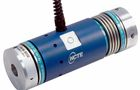# Conveyor Belt Torque Calculation

- Dec 21, 2018-

Torque is a special kind of torque that causes an object to rotate. The torque of the engine is the torque that the engine outputs from the crankshaft end. It is inversely proportional to the engine speed under the condition of fixed power. The faster the speed, the smaller the torque, and vice versa, it reflects the load capacity of the car within a certain range.

The external torque is called torque or external force moment, and the internal one is called internal torque or torque.Basic Information

Chinese name:Torque

Foreign name:Torque

Force and:Engine torque

Pound ruler:Crankshaft output

product:External force momentFormula calculation

Overall, the driving force of a car can be calculated by the following formula:

formula

Driving force=torque×transmission gear ratio×final gear ratio×mechanical efficiency÷tire radius (unit: meter)

Summary: 1kgm=9.8Nm1lb-ft=0.13826kgm1lb-ft=1.355Nm

In the case of a certain displacement, the cylinder diameter is small, and the cylinder with a long stroke pays more attention to the torque, and the rotation speed is not too high, and is suitable for a vehicle that requires a large load. The cylinders with large bore diameter and short stroke are more focused on power output, and the rotational speed is usually higher, which is suitable for fast running vehicles. Simply put: power is proportional to torque × speed.

Calculation

Why is the power of the engine calculated from the torque?

Power P = work W ÷ time t work W = force F × distance s, so P = F × s / t = F × speed v

Here v is the linear velocity, and in the engine, the linear velocity of the crankshaft = the angular velocity of the crankshaft ω × the crankshaft radius r, substituted into the above formula: power P = force F × radius r × angular velocity ω; and force F × radius r = Torque

It is concluded that the power P = torque × angular velocity ω so the power of the engine can be calculated from the torque and the rotational speed.

The unit of angular velocity is radians/second. In the arc system, a π represents 180 degrees.